1. /
2. CBSE
3. /
4. Class 08
5. /
6. Mathematics
7. /
8. NCERT Solutions for Class...

# NCERT Solutions for Class 8 Maths Exercise 6.4### myCBSEguide App

Download the app to get CBSE Sample Papers 2023-24, NCERT Solutions (Revised), Most Important Questions, Previous Year Question Bank, Mock Tests, and Detailed Notes.

NCERT solutions for Class 8 Maths Squares and Square Roots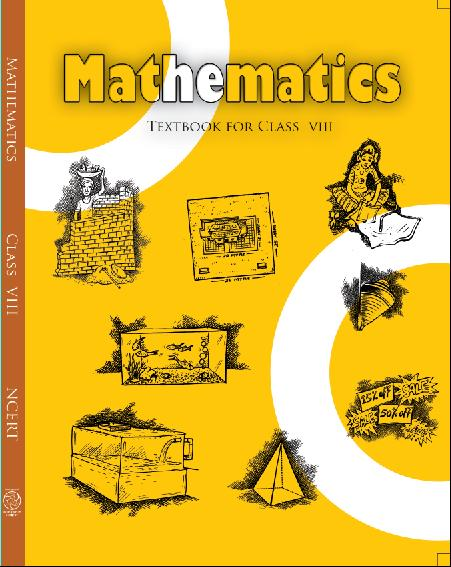## NCERT Solutions for Class 8 Maths Squares and Square Roots

###### 1. Find the square roots of each of the following numbers by Division method:

(i) 2304     (ii) 4489

(iii) 3481     (iv) 529

(v) 3249     (vi) 1369

(vii) 5776     (viii) 7921

(ix) 576     (x) 1024

(xi) 3136     (xii) 900

Ans. (i) 2304

Hence, the square root of 2304 is 48.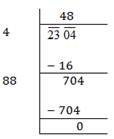(ii) 4489

Hence, the square root of 4489 is 67.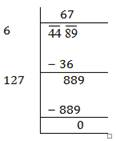(iii) 3481

Hence, the square root of 3481 is 59.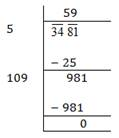(iv) 529

Hence, the square root of 529 is 23.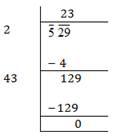(v) 3249

Hence, the square root of 3249 is 57.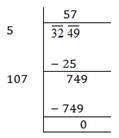(vi) 1369

Hence, the square root of 1369 is 37.(vii) 5776

Hence, the square root of 5776 is 76.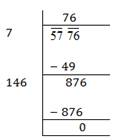(viii) 7921

Hence, the square root of 7921 is 89.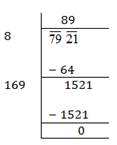(ix) 576

Hence, the square root of 576 is 24.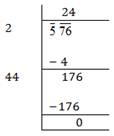(x) 1024

Hence, the square root of 1024 is 32.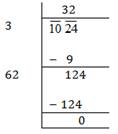(xi) 3136

Hence, the square root of 3136 is 56.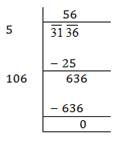(xii) 900

Hence, the square root of 900 is 30.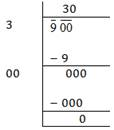###### 2. Find the number of digits in the square root of each of the following numbers (without any calculation):

(i) 64

(ii) 144

(iii) 4489

(iv) 27225

(v) 390625

Ans. (i) Here, 64 contains two digits which is even.

Therefore, number of digits in square root =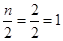(ii) Here, 144 contains three digits which is odd.

Therefore, number of digits in square root =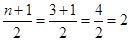(iii) Here, 4489 contains four digits which is even.

Therefore, number of digits in square root =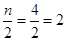(iv) Here, 27225 contains fivr digits which is odd.

Therefore, number of digits in square root =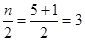(v) Here, 390625 contains six digits which is even.

Therefore, number of digits in square root =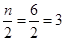NCERT Solutions for Class 8 Maths Exercise 6.4

###### 3. Find the square root of the following decimal numbers:

(i) 2.56

(ii) 7.29

(iii) 51.84

(iv) 42.25

(v) 31.36

Ans. (i) 2.56

Hence, the square root of 2.56 is 1.6.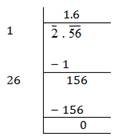(ii) 7.29

Hence, the square root of 7.29 is 2.7.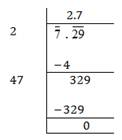(iii) 51.84

Hence, the square root of 51.84 is 7.2.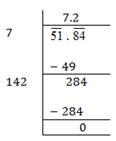(iv) 42.25

Hence, the square root of 42.25 is 6.5.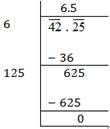(v) 31.36

Hence, the square root of 31.36 is 5.6.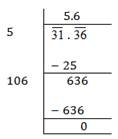NCERT Solutions for Class 8 Maths Exercise 6.4

###### 4. Find the least number which must be subtracted from each of the following numbers so as to get a perfect square. Also, find the square root of the perfect square so obtained:

(i) 402

(ii) 1989

(iii) 3250

(iv) 825

(v) 4000

Ans. (i) 402

We know that, if we subtract the remainder from the number, we get a perfect square.

Here, we get remainder 2. Therefore 2 must be subtracted from 402 to get a perfect square.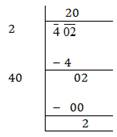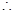402 – 2 = 400

Hence, the square root of 400 is 20.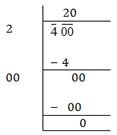(ii) 1989

###### We know that, if we subtract the remainder from the number, we get a perfect square.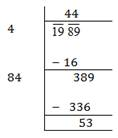Here, we get remainder 53. Therefore 53must be subtracted from 1989 to get a perfect square.1989 – 53 = 1936

Hence, the square root of 1936 is 44.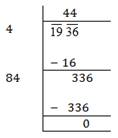(iii) 3250

We know that, if we subtract the remainder from the number, we get a perfect square.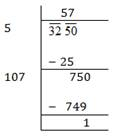Here, we get remainder 1. Therefore 1 must be subtracted from 3250 to get a perfect square.3250 – 1 = 3249

Hence, the square root of 3249 is 57.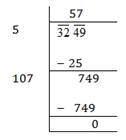(iv) 825

We know that, if we subtract the remainder from the number, we get a perfect square.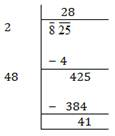Here, we get remainder 41. Therefore 41 must be subtracted from 825 to get a perfect square.825 – 41 = 784

Hence, the square root of 784 is 28.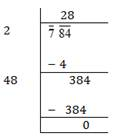(v) 4000

We know that, if we subtract the remainder from the number, we get a perfect square.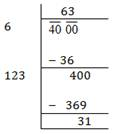Here, we get remainder 31. Therefore 31 must be subtracted from 4000 to get a perfect square.4000 – 31 = 3969

Hence, the square root of 3969 is 63.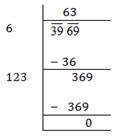NCERT Solutions for Class 8 Maths Exercise 6.4

###### 5. Find the least number which must be added to each of the following numbers so as to get a perfect square. Also, find the square root of the perfect square so obtained:

(i) 525

(ii) 1750

(iii) 252

(iv) 1825

(v) 6412

Ans. (i) 525

Since remainder is 41.

Therefore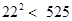Next perfect square number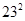= 529

= 529 – 525 = 4525 + 4 = 529

Hence, the square root of 529 is 23.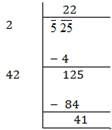(ii) 1750

Since remainder is 69.

Therefore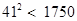Next perfect square number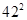= 1764

= 1764 – 1750 = 141750 + 14 = 1764

Hence, the square root of 1764 is 42.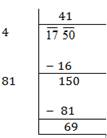(iii) 252

Since remainder is 27.

Therefore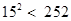Next perfect square number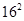= 256

= 256 – 252 = 4252 + 4 = 256

###### Hence, the square root of 256 is 16.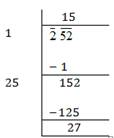(iv) 1825

Since remainder is 61.

Therefore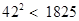Next perfect square number= 1849

Hence, number to be added = 1849 – 1825 = 241825 + 24 = 1849

Hence, the square root of 1849 is 43.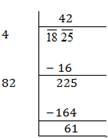(v) 6412

Since remainder is 12.

ThereforeNext perfect square number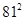= 6561

= 6561 – 6412 = 1496412 + 149 = 6561

Hence, the square root of 6561 is 81.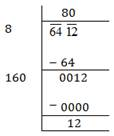NCERT Solutions for Class 8 Maths Exercise 6.4

###### 6. Find the length of the side of a square whose area is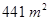?

Ans. Let the length of side of a square be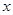meter.

Area of square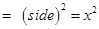According to question,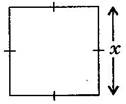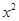= 441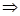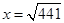=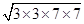=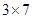= 21 m

Hence, the length of side of a square is 21 m.

NCERT Solutions for Class 8 Maths Exercise 6.4

###### 7. In a right triangle ABC,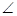B =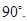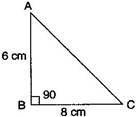(i) If AB = 6 cm, BC = 8 cm, find AC.

(ii) If AC = 13 cm, BC = 5 cm, find AB.

Ans. (a) Using Pythagoras theorem,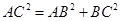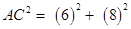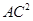= 36 + 84 = 100AC = 10 cm

(b) Using Pythagoras theorem,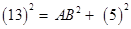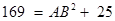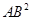= 169 – 25= 144AB= 12 cm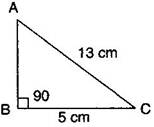NCERT Solutions for Class 8 Maths Exercise 6.4

###### 8. A gardener has 1000 plants. He wants to plant these in such a way that the number of rows and number of columns remain same. Find the minimum number of plants he needs more for this.

Ans. Here, plants = 1000

Since remainder is 39.

Therefore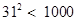Next perfect square number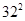= 1024

= 1024 – 1000 = 241000 + 24 = 1024

Hence, the gardener required 24 more plants.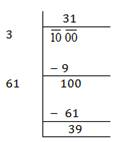NCERT Solutions for Class 8 Maths Exercise 6.4

###### 9. There are 500 children in a school. For a P.T. drill they have to stand in such a manner that the number of rows is equal to number of columns. How many children would be left out in this arrangement?

Ans. Here, Number of children = 500

By getting the square root of this number, we get,

In each row, the number of children is 22.

And left out children are 16.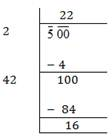## NCERT Solutions for Class 8 Maths Exercise 6.4

NCERT Solutions Class 8 Mathematics PDF (Download) Free from myCBSEguide app and myCBSEguide website. Ncert solution class 8 Mathematics includes text book solutions from Class 8 Maths Book . NCERT Solutions for CBSE Class 8 Maths have total 16 chapters. 8 Maths NCERT Solutions in PDF for free Download on our website. Ncert class 8 solutions PDF and Maths ncert class 8 PDF solutions with latest modifications and as per the latest CBSE syllabus are only available in myCBSEguide.

## CBSE app for Class 8

To download NCERT Solutions for class 8 Social Science, Computer Science, Home Science,Hindi ,English, Maths Science do check myCBSEguide app or website. myCBSEguide provides sample papers with solution, test papers for chapter-wise practice, NCERT solutions, NCERT Exemplar solutions, quick revision notes for ready reference, CBSE guess papers and CBSE important question papers. Sample Paper all are made available through the best app for CBSE students and myCBSEguide website.Test Generator

Create question paper PDF and online tests with your own name & logo in minutes.myCBSEguide

Question Bank, Mock Tests, Exam Papers, NCERT Solutions, Sample Papers, Notes

### 26 thoughts on “NCERT Solutions for Class 8 Maths Exercise 6.4”

1. Nice

2. Thank you for clear my dout this is good way for study

3. Thanks , You are great .

4. Good

5. Nice

7. THE ANSWER IS TRUELY RIGHT
HOW YOU SOLVE THIS PROBLEM

8. Nice

9. THANK YOU FOR THIS SOLUTION

11. Nice

12. Very nice thanks

13. It’s really helpful to me..

14. Thanks for a big help by these answer. I have really received a good help .
Thanks a lot by my heart.
???

15. Nice

16. Thanks a lot I am very happy super

17. Now my doubt is clear now Thank You

18. Good

19. Thanks a lot by my heart

20. Good very nice to patient

21. Nice preparation

22. Thanks very useful

23. Thanks for this site?. I was just frustrated with the sums?

24. Thankyou so much !! Its very useful to me

25. Thanks to clear my doubt… It’s good

26. thanks it’s good